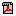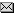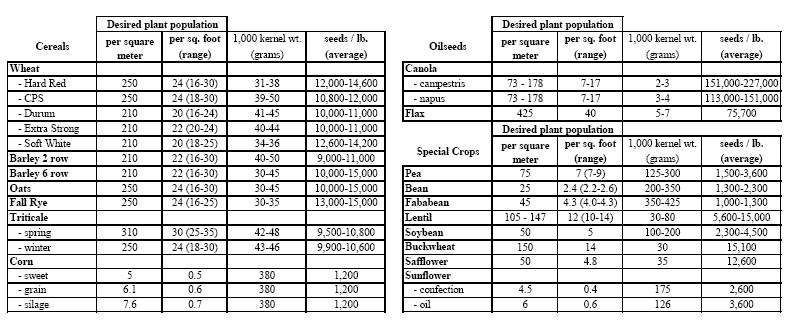# Calculator for Estimating Crop YieldPDF(100K)Agri-NewsThis Week

A calculation procedure to assist you to estimate your crop yield is available at Using 1,000 Kernel Weight for Calculating Seeding Rates and Harvest Losses.

In estimating the yield of a crop, the producer would be counting the total number of viable kernels in a known area (foot2). These are then weighed, or a standard 1,000 kernel weight can be used. This can be extrapolated to an acreage level to give an estimate of potential crop yield.

Estimating crop yield
Yield (in kg/acre*) can be estimated using the following formula:

 Yield = Heads/ft2 x seeds/head x x '1,000 kernel weight' x x 0.43560
Using an HRS wheat example with estimates of 26 heads/ft2 , 24 seeds/head and the '1,000 kernel weight of 35 grams (from the table), the yield works out to: = 26 heads/ft2 x 24 seeds/head x x 35 x 0.043560 = 951.4 kg/acre Given wheat at 27.215 kg/bu, the estimated yield would be about 35 bu./ac.

The logic for the calculation is provided below:

Grams per acre = (Kernels per acre x kernel weight) / 1,000

Kg/ac = grams per acre / 1,000

*-AFSC uses kg/ac for insurance purposes